# Bulk Modulus,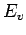Bulk Modulus determines how compressible a fluid is. In other words, it tells us how the density of a fluid changes when the fluid is subjected to pressure. It is defined as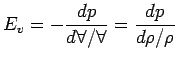(1.19)

where dp is the pressure change that is required in order to change the volume by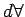,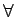being the initial volume. The negative sign indicates that an increase in pressure is accompanied by a decrease in volume.

The dimensions of Bulk Modulus are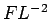; the units being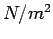. Higher the value of Bulk Modulus indicates that it is difficult to compress the fluid. Take water for example. Its Bulk Modulus is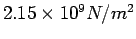meaning that it requires an enormous pressure to change the volume of water by a small amount. For all practical purposes water (and many other liquids as well) is treated as being incompressible.

Subsections

(c) Aerospace, Mechanical & Mechatronic Engg. 2005
University of Sydney